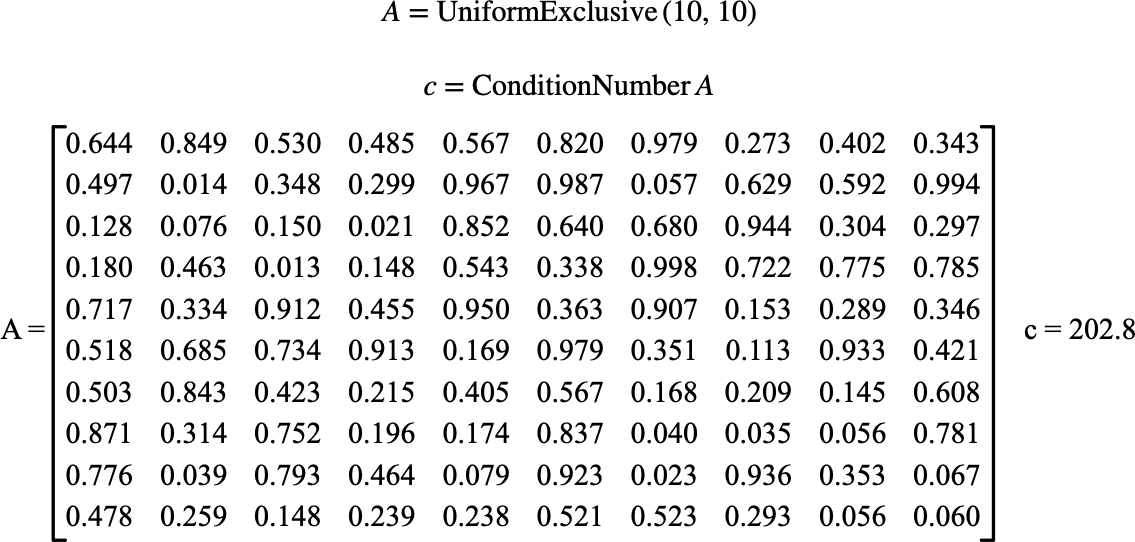# $$\text{ConditionNumber}$$¶

You can use the $$\text{ConditionNumber}$$ function to calculate an approximate condition number of a matrix.

You can use the \condnum backslash command to insert this function.

The following variants of this function are available:

• $$\text{real } \text{ConditionNumber} \left ( \text{<matrix>} \right )$$

The function calculates the approximate condition number using the relation:

$\text{ConditionNumber} \left ( A \right ) = \left \Vert A \right \Vert _ 2 \left \Vert A ^ {-1} \right \Vert _ 2$

Where $$\left \Vert X \right \Vert _ 2$$ represents the entry-wise Euclidean norm of the matrix.

Note

Given the computational complexity in calculating the condition number, future versions of Aion may modify the underlying implementation to improve performance at the expense of accuracy.

The function will produce a run-time error or return NaN if the provided matrix is singular.

Figure 121 demonstrates the basic use of the $$\text{ConditionNumber}$$ function.Figure 121 Example Use Of the ConditionNumber Function